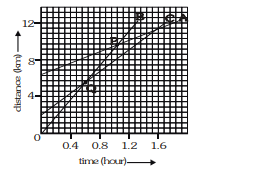# Figure below shows the distance-time graph of three objectsQuestion.
Figure below shows the distance-time graph of three objects A, B and C. Study the graph and answer the following questions :
(a) Which of the three is travelling the fastest ?
(b)Are all three ever at the same point on the road?
(c) How far has C travelled when B passes A ?
(d) How far has B travelled by the time it passes C ?Solution:
(a) Car B is travelling the fastest, because its slope is largest among the three.
(b) No, they are never at the same point because all the graphs of $\mathrm{A}, \mathrm{B}$ and $\mathrm{C}$ do not intersect at one point.
(c) When car B passes car A at point P, the distance covered by car C $=8-2=6 \mathrm{~km}$. (approx.)
(d) Car B and C pass each other at point Q. The distance travelled by B at that point is nearly $5.7 \mathrm{~km}$.